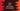# Find ASCII value of a Character in Java## Write a program in Java to find ASCII value of a Character :

ASCII or American Standard Code for Information Interchange is a character encoding standard to represent characters in computers. By using a 7-bit binary number, a character is defined.

There are 128 possible characters. In decimal form, we can represent a character from 0 to 127. In this tutorial, I will show you how to get the decimal ASCII value of a character in Java.

To find ASCII value, we have two different ways :

1. Assign the character to an integer variable’
2. Typecast the character to an integer.

Below program explains both approaches :

``````public class Test {
public static void main(String[] args) {

Character c = 'b';

int value = c;

//1 : Assigning
System.out.println("Ascii : "+value);

//2 : Typecasting
System.out.println("Ascii : "+(int)c);
}
}``````

### Output :

``````Ascii : 98
Ascii : 98``````